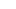# Hands-On Multiplication Puzzles Activity – FREE Printable

If you are looking for a fun way of teaching multiplication, these multiplication printables are a fun way to introduce your kids to the concept of multiplication. These multiplication activity can be used to introduce your children to the concept of multiplication using repeated addition of equal groups. Simple download the multiplication printables pdf file and great ready make learning multiplication for 2nd grade, 3rd grade, and 4th grade students FUN!## Multiplication Puzzles

Once kids learn about counting in groups or skip counting, they are ready to ease into this easy way to teach multiplication. As you are teaching multiplication, it is improtant to give kids a lot of practice to make the concept click. And although multiplication worksheets are a easy way to do that, they are not as engaging for kids. Use these multiplication puzzles as a fun, free multiplication printable to make math fun for grade 2, grade 3, and grade 4 students. Whether you are a parent, teacher, or homeschooler – you will love this hands-on multiplication activity.

## Multiplication printables

Start by scrolling to the bottom of the post, under the terms of use, and click on the text link that says >> Download <<. The pdf file will open in a new window for you to save the freebie and print the template.## Teaching Multiplication

I love teaching maths in general because it is a subject that can be taught using many visual and hands on aids. These aids can be using manipulatives such as base 10 blocks or hands on math stations and centers. In this post, I will be sharing an interactive hands on printable which can be used to teach multiplication and is an ideal addition into math centers. Hopefully, this printable will help to make teaching multiplication at your home or classroom more enjoyable.

To start off, there are a few models that can be used to teach multiplication.

1. Equal groups
2. Arrays
4. Number line

## Introducing Multiplication

When teaching multiplication it is important that children get the concept before just memorizing the multiplication facts. This is so that they are able to apply these concepts into their daily lives (for e.g. in counting money). Multiplication can be first introduced using the model of repeated addition of equal groups. This makes sense to me, because since children are already very familiar with the concept of addition, they can use the concept of addition and expand it out to the concept of multiplication.

As students learn their multiplication facts through the repeated addition they will begin to realize how multiplication is much easier than addition. Rather than having to write out long addition statements they can just shorten it into a simple multiplication fact. This is very obvious in these multiplication fact booklets, where the number of blanks for the repeated addition equations keeps on increasing depending on number of groups.## Easy way to teach multiplication

To make things a little bit more fun, I created some multiplication matching puzzles where they have to match the visual representation of equal groups to the multiplication and repeated addition representation as well as the total number. This is an ideal hands-on practice to accompany the concept of concept of multiplication through repeated addition of equal groups. The printable contains puzzles only until facts of 5, since this activity is only meant to work as an introduction to multiplication.

In this printable, each puzzle has 4 pieces. Students need to match up the 4 pieces for each multiplication concept. Students can paste the completed puzzles on a paper or it can be laminated and re-used if its for a math center. The puzzle templates are easy cut, so these are relatively easy to prep. The puzzles come in colour and black and white, so just print which version suits you.## Multiplication Activity

### To Use:

• Print the file either in colour or black and white and laminate (this is recommended, if you are planning to re-use the puzzles)
• Cut apart the puzzle along the borders to separate it into 4 equal pieces.

### Presentation:

• Present the puzzle pieces to the students. If the student is extremely new to the concept, introduce only one puzzle at a time.
• Ask them to fit in the different methods of representing the equal groups (multiplication equation, repeated addition equation and the total).

Try doing a few puzzles together with them, to let them understand the concept and what they are required to do. Start of by explaining that the groups shown are equal with each group having the same number of items (e.g. 4 bees in each hive, 5 balloons in each sky group, and so on). Now, we are ready to start solving the puzzle and matching the pieces.

• Next, ask them to count the number of items in each group.
• Then ask them to form an addition sentence, and to find the piece of the puzzle that matches it.
• Add it together, to find the total.
• Finally, help them to construct the multiplication statement and solve the whole puzzle.

This multiplication math activity, when done a few times will help to set a basic foundation of multiplication. This will make exploring multiplication facts easier. It will also make it easier to teach the other models of multiplication such as arrays and number lines.

## Multiplication game printable

• This is for personal use only (teachers please see my TPT store)
• This may NOT be sold, hosted, reproduced, or stored on any other site (including blog, Facebook, Dropbox, etc.)
• Graphics purchased and used with permission
• I offer free printables to bless my readers AND to provide for my family. Your frequent visits to my blog & support purchasing through affiliates links and ads keep the lights on so to speak. Thanks you!•Terry says:
•Beth Gorden says: